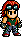# Emily Rochefort/Tekken 5: Dark Resurrection MovelistLooks like Hwoarang has run off and made this page, Emily Rochefort/Tekken 5: Dark Resurrection Movelist, without telling Baek Doo San and hasn't done it right!Please fix it by re-formatting this page to follow it's template.

Emily Rochefort/Tekken 5: Dark Resurrection Movelist is a list of all of the moves that Lili can perform in Tekken 5: Dark Resurrection.

## §Moves List

1. Left Right Back Strike: Square Triangle X.
2. Left Right Piercing Thorn: Square Triangle Circle.
3. Sunrise Sunset: Triangle X.
4. Bed Time: Triangle Circle.
5. Broken Heart: Square + Triangle.
6. Divine Step: X + Circle.
7. Pendulum Strike: East Triangle Square + Triangle.
8. Circle Knee: East X.
9. Ivory Revolver: East Circle.
10. Stinging Nettle: East Square + Triangle.
11. Sundial: East X + Circle.
12. Delayed Sundial: East X + Circle X.
13. Garland Kick Combo: East X + Circle X Circle.
14. Direct Persuasion: Southeast Square.
15. Submissive Heel: Southeast X.
16. Dominating Heel: Southeast Circle Circle.
17. Backflip Spinning Edge: Southeast X + Circle X + Circle.
18. Heavy Languish: South Square Triangle Circle.
19. Bloody Masquerade Piton: South Triangle Triangle X.
20. Bloody Masquerade Lance: South Triangle Triangle Circle.
21. Matterhorn Ascension: South X + Circle.
22. Freesia Thrust: Southwest X.
23. Edelweiss: Southwest Circle.
24. Shattered Crown: West Square.
25. Heliotrope: West Triangle Square.
26. Twist of Fate: West X.
27. Feisty Rabbit: West Circle.
28. Rabbit's Foot: West [Circle X].
29. Rabbit Thorn: West [Circle Circle].
30. Dendrobium: West Square + Triangle.
31. Mirror Image: West X + Circle.
32. Piercing Thorn Fortissimo: West Square + Circle.
33. Angel Knee: Northeast X + Circle.
34. Angel Knee Ascension: Northeast X + Circle X + Circle.
35. Sunflower Lance: Northeast Circle X.
36. Allonger Le Bras: East East (hold) Triangle.
37. Alstroemeria: East East (hold) X X + Circle.
38. Root of Evil: East East (hold) Circle.
39. Piercing Thorn: East East (hold) X + Circle.
40. Dew Glide: South Southeast East.
41. Slashing Vine: South Southeast East Square.
42. Angel Heart: South Southeast East Triangle.
43. En Garde: (While rising) Square.
44. Delicate Uprising: (While rising) Triangle.
45. Honeysuckle: (While rising) X Circle.
46. Horizon Slide: (While crouching) Southeast X.
47. Stunning Toe Kick: (During sidestep) X.
48. Minuet: (During sidestep) Square + Triangle.
49. Shears to Spinning Edge: (Back towards enemy) Circle X + Circle.
50. Tempest: (Back towards enemy) Square + Triangle.
51. Reverse Divine Step: (Back towards enemy) X + Circle.
52. Autumn Wind: (Back towards enemy) South X Circle.
53. Orchid Ring: (Back towards enemy) South X + Circle.
54. 10 Hit Combo 1: Circle Square X Circle Circle X Circle Circle X + Circle.
55. 10 Hit Combo 2: Circle Square X X + Circle X Circle X Square X + Circle Circle.
56. 10 Hit Combo 3: Circle Square X X + Circle.
57. Kiss of Devotion: (Approach enemy) Square + X (or East Square + X).
58. Femme Fatale: (Approach enemy) Triangle + Circle (or East Triangle + Circle).
59. Soleil: (Approach from left side) Square + X (or Triangle + Circle).
60. Monaco Mixer: (Approach from right side) Square + X (or Triangle + Circle).
61. Ravaging Beauty: (Approach from behind) Square + X (or Triangle + Circle).
62. Rafflesia: (Approach enemy) Southeast Square + Triangle.
63. Attack Reversal: (Time with enemy punch) West Square + X (or West Triangle + Circle).